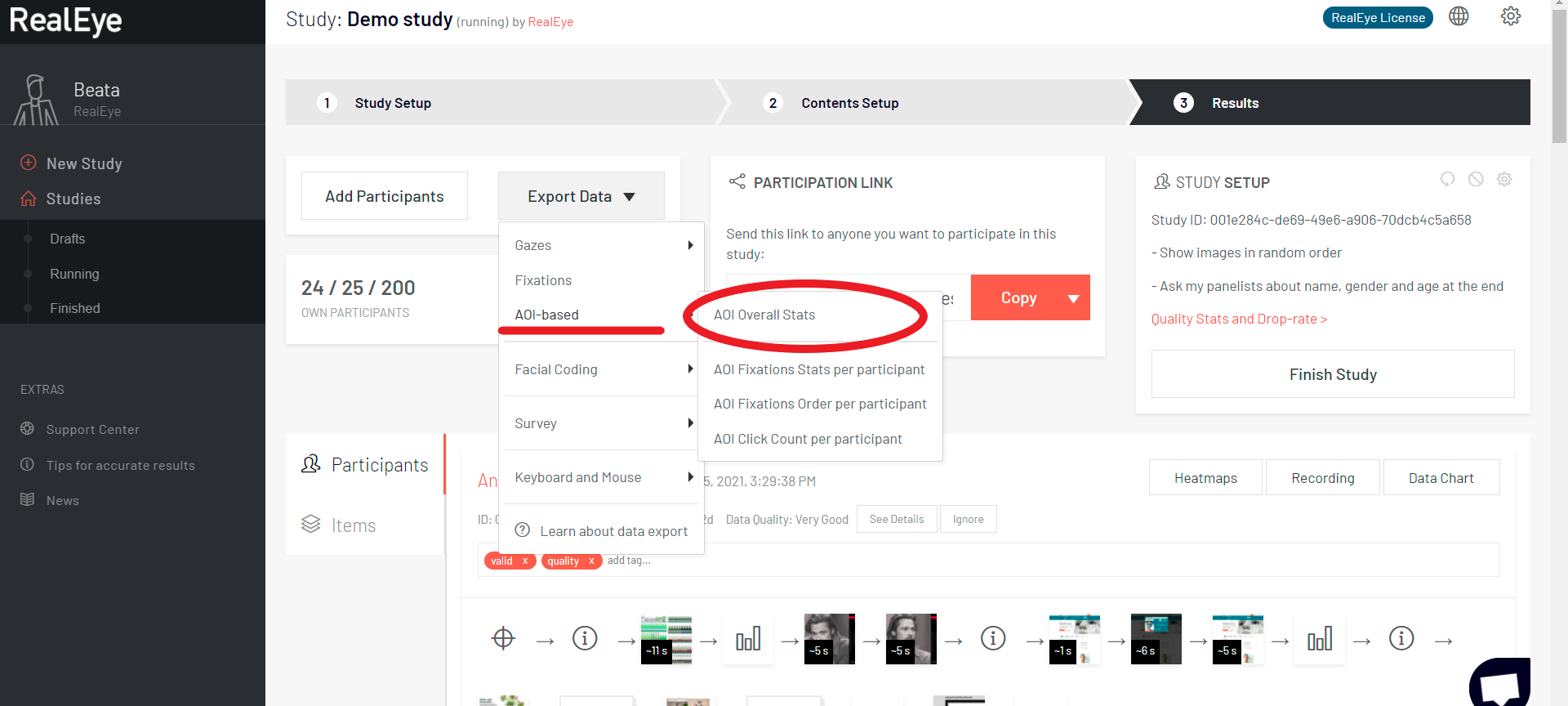CSV file: AOI overall statsThe "AOI overall stats" CSV contains the same metrics that are included in AOI on the heatmap dashboard. There are the following fields:

• string study_id - UUID,
• string item_id - UUID,
• string item_filename - the name of your file, as it was named while adding to study,
• string item_cdn_url - URL to the tested item saved on our servers,
• int tester_count - for how many participants the eye-tracking data was collected for this item,
• string aoi_id - UUID,
• string aoi_name - the name that you give to an AOI while saving it,
• string aoi_item_parts - exact AOI parameters (area and time range), especially important for multi AOI,
• float aoi_size_overall_percents - expressed as the percentage value relative to the size of the entire image,
• int aoi_time_range_from_ms - the start time from which the values of the AOI were calculated (you can set this time with the orange slider under the heatmap),
• int aoi_time_range_to_ms - the end time to which the values of the AOI were calculated (you can set this time with the orange slider under the heatmap),
• int aoi_fixation_total_count - number of fixations within this area,
• int aoi_fixation_average_ttff_ms - the amount of time that it takes participants (on average) to make the first fixation at this area (in milliseconds),
• int aoi_fixation_average_total_time_spent_ms - the average amount of time that all the participant's fixations lasted in total (in milliseconds),
• int aoi_fixation_average_fixation_duration_ms - the time that the average fixation lasted for (in milliseconds),
• int aoi_fixation_average_first_fixation_duration_ms - the time that the average first fixation lasted for (in milliseconds),
• int aoi_fixation_tester_ratio_percents - how many of all the participants looked at the given area (based on fixations),
• int aoi_gaze_total_count - number of gazes within this area,
• int aoi_gaze_average_time_to_first_gaze_at_ms - the amount of time that it takes participants (on average) to look at this area (in milliseconds),
• int aoi_gaze_average_total_time_spent_ms - the average amount of time that a participant looked at the area based on gazes (in milliseconds),
• int aoi_gaze_total_time_spent_ms - the total amount of time that all participants looked at the area based on gazes (in milliseconds),
• float aoi_gaze_tester_ratio_percents - how many of all the participants looked at the given area (based on gazes),
• int aoi_visit_total_count - AOI visit is detected if there's a fixation within the AOI (or many fixations "one after another"),
• int aoi_revisit_total_count - how many times participants looked back at the AOI (in total),
• float aoi_revisit_average_count - how many times participants looked back at the AOI (on average),
NOTE:
If the participant didn't looked at the AOI, then visits = 0, revisits = 0.
If the participant looked once at the AOI, then visits = 1, revisits = 0.
If the participant looked N-times at the AOI, then visits = N, revisits = N-1.
The " average revisits count takes into the account those who have not looked at the AOI (it includes those with 0 visits and revisits).
We count separate visit if there's a long data gap between fixations.

• int aoi_click_total_count - how many clicks all participants made within this AOI,
• int aoi_click_average_time_to_first_click_ms - the amount of time that it takes participants (on average) to click at this area for the first time,
• int aoi_word_total_count - how many words were registered within the area.
• notes - this column contains:
• RealEye version,
• fixation filter settings:
• gazepoint interpolation (Hz) - to improve calculations we interpolate the data to 60 Hz;
• gazepoint interpolation max gap (ms) - we interpolate data if the timestamp gap between two input values is not bigger than this value (50 ms);
• gaze velocity threshold;
• min. fixation duration (Ms);
• noise reduction level,
• isGenderMale={true/false},
• isGenderFemale={true/false},
• isGenderOther={true/false},
• isGenderUnknown={true/false},
• qualityMin={from1 to 6},
• qualityMax={from 1 to 6},
• isTesterTypeRealEye={true/false},
• isTesterTypeNonRealEye={true/false},